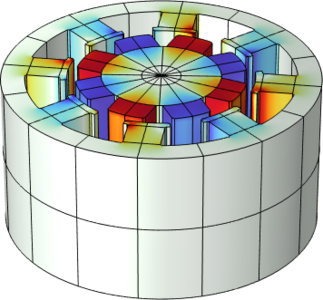# Application Gallery

#### Modeling of an Electric Generator in 3D

Application ID: 20191

These models demonstrate how to set up a sector model of rotating machinery both in 2D and 3D using the Rotating Machinery, Magnetic interface in COMSOL Multiphysics®. The stator in these permanent magnet AC generator examples consists of a stator winding backed by an iron core. The rotor includes the permanent magnets and iron core.

The 2D and 3D models of the generator are included here. In 2D, the following models are included:

• A full 2D cross-section model with quadratic mesh elements and a nonlinear B-H/H-B curve
• Two 1/8th sector models with quadratic mesh elements and a nonlinear B-H/H-B curve

The corresponding 3D sector models include a combination of linear and quadratic mesh elements and linear and nonlinear B-H/B-H curves. The rotor is modeled using magnetic scalar potential and the stator using magnetic vector potential.

• Linear mesh elements and linear materials
• Linear mesh elements and nonlinear B-H/H-B curve
• Quadratic mesh elements and nonlinear B-H/H-B curve

This model is featured and explained in much greater detail in the following blog post: Guidelines for Modeling Rotating Machines in 3D.This model example illustrates applications of this type that would nominally be built using the following products: